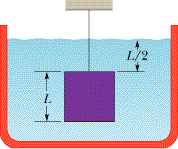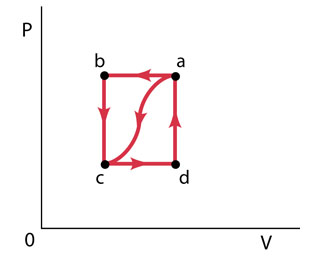Ask question

# a) Find the function's domain . b) Find the function's range. c) Find the boundary of the function's domain. d) Determine if the domain is an open region, a closed region , or neither. e) Decide if the domain is bounded or unbounded. for: f(x,y) = 1/((16- x^2 -y^2)^(1/2))# a) Find the function's domain . b) Find the function's range. c) Find the boundary of the function's domain. d) Determine if the domain is an open region, a closed region , or neither. e) Decide if the domain is bounded or unbounded. for: f(x,y) = 1/((16- x^2 -y^2)^(1/2))

Question
Multivariable functionsasked 2020-11-02
a) Find the function's domain .
b) Find the function's range.
c) Find the boundary of the function's domain.
d) Determine if the domain is an open region, a closed region , or neither.
e) Decide if the domain is bounded or unbounded.
for: $$\displaystyle{f{{\left({x},{y}\right)}}}=\frac{{1}}{{{\left({16}-{x}^{{2}}-{y}^{{2}}\right)}^{{\frac{{1}}{{2}}}}}}$$

## Answers (1)2020-11-03
a)Determine the domain of the multivariable function.
$$\displaystyle{f{{\left({x},{y}\right)}}}=\frac{{1}}{\sqrt{{{16}-{x}^{{2}}-{y}^{{2}}}}}$$
$$\displaystyle{16}-{x}^{{2}}-{y}^{{2}}\ge{0}$$
$$\displaystyle-{x}^{{2}}-{y}^{{2}}\ge-{16}$$
$$\displaystyle-{1}\times{\left(-{x}^{{2}}-{y}^{{2}}\right)}\le-{1}\times-{16}$$
$$\displaystyle{x}^{{2}}+{y}^{{2}}\le{16}$$
Also, $$\displaystyle\sqrt{{{16}-{x}^{{2}}-{y}^{{2}}}}{>}{0}$$
$$\displaystyle{16}-{x}^{{2}}-{y}^{{2}}{>}{0}$$
$$\displaystyle{x}^{{2}}+{y}^{{2}}{<}{16}$$</span>
From, $$\displaystyle{x}^{{2}}+{y}^{{2}}\le{16}{\quad\text{and}\quad}{x}^{{2}}+{y}^{{2}}{<}{16}$$</span>
The domain for f(x,y) is (x,y) $$\displaystyle\in\mathbb{R}^{{2}}:{x}^{{2}}+{y}^{{2}}{<}{16}.$$</span>
b) Evaluate the range for the given function.
Range for $$\displaystyle{16}−{x}^{{2}}−{y}^{{2}}{i}{s}{\left({0},{16}\right]}.$$
Therefore, range for f(x,y) is
$$\displaystyle{f{{\left({x},{y}\right)}}}=\frac{{1}}{\sqrt{{{16}-{x}^{{2}}-{y}^{{2}}}}}$$
As $$\displaystyle{16}−{x}^{{2}}−{y}^{{2}}\to{0},{f{{\left({x},{y}\right)}}}\to\infty$$
As $$\displaystyle{16}−{x}^{{2}}−{y}^{{2}}\to{16},{f{{\left({x},{y}\right)}}}\to{14}$$
Therefore, range for $$\displaystyle{f{{\left({x},{y}\right)}}}\in{\left[\frac{{1}}{{4}},\infty\right)}.$$
c)Determine the boundary of the domain using the domain for f(x, y).
The domain for f(x,y) is (x,y) $$\displaystyle\in\mathbb{R}^{{2}}:{x}^{{2}}+{y}^{{2}}{<}{16}.$$</span>
The equation $$\displaystyle{x}^{{2}}+{y}^{{2}}{<}{16}$$</span> is a circle.
The boundary for the domain is points lying in the circle $$\displaystyle{x}^{{2}}+{y}^{{2}}={16}.$$
d) The domain for the function f(x, y) do not contain the boundary points. Therefore, the domain for the function is an open region.
e) The domain for the function is enclosed in the circle.
Hence, the domain is bounded in the circle of equation $$\displaystyle{x}^{{2}}+{y}^{{2}}{<}{16}.$$</span>

### Relevant Questionsasked 2021-05-05
The bulk density of soil is defined as the mass of dry solidsper unit bulk volume. A high bulk density implies a compact soilwith few pores. Bulk density is an important factor in influencing root development, seedling emergence, and aeration. Let X denotethe bulk density of Pima clay loam. Studies show that X is normally distributed with $$\displaystyle\mu={1.5}$$ and $$\displaystyle\sigma={0.2}\frac{{g}}{{c}}{m}^{{3}}$$.
(a) What is thedensity for X? Sketch a graph of the density function. Indicate onthis graph the probability that X lies between 1.1 and 1.9. Findthis probability.
(b) Find the probability that arandomly selected sample of Pima clay loam will have bulk densityless than $$\displaystyle{0.9}\frac{{g}}{{c}}{m}^{{3}}$$.
(c) Would you be surprised if a randomly selected sample of this type of soil has a bulkdensity in excess of $$\displaystyle{2.0}\frac{{g}}{{c}}{m}^{{3}}$$? Explain, based on theprobability of this occurring.
(d) What point has the property that only 10% of the soil samples have bulk density this high orhigher?
(e) What is the moment generating function for X?asked 2021-05-16
Consider the curves in the first quadrant that have equationsy=Aexp(7x), where A is a positive constant. Different valuesof A give different curves. The curves form a family,F. Let P=(6,6). Let C be the number of the family Fthat goes through P.
A. Let y=f(x) be the equation of C. Find f(x).
B. Find the slope at P of the tangent to C.
C. A curve D is a perpendicular to C at P. What is the slope of thetangent to D at the point P?
D. Give a formula g(y) for the slope at (x,y) of the member of Fthat goes through (x,y). The formula should not involve A orx.
E. A curve which at each of its points is perpendicular to themember of the family F that goes through that point is called anorthogonal trajectory of F. Each orthogonal trajectory to Fsatisfies the differential equation dy/dx = -1/g(y), where g(y) isthe answer to part D.
Find a function of h(y) such that x=h(y) is the equation of theorthogonal trajectory to F that passes through the point P.asked 2021-04-27
Let $$\displaystyle{f{{\left({x},{y}\right)}}}={x}^{{2}}{e}^{{{3}{x}{y}}}$$ (a) evaluate f(2,0) (b) find the domain of f (c) find the range of f.asked 2021-04-22
The graph of f '' is give below. Draw graphs of f and f ',assuming both go through the origin, and use them to decide atwhich of the labeled x-values:
a) f(x) is greatest.
b) f(x) is least.
c) f '(x) is greatest.
d) f '(x) is least.
e) f '' (x) is greatest.
f) f '' (x) is least.asked 2021-03-20
The graph of y = f(x) contains the point (0,2), $$\displaystyle{\frac{{{\left.{d}{y}\right.}}}{{{\left.{d}{x}\right.}}}}={\frac{{-{x}}}{{{y}{e}^{{{x}^{{2}}}}}}}$$, and f(x) is greater than 0 for all x, then f(x)=
A) $$\displaystyle{3}+{e}^{{-{x}^{{2}}}}$$
B) $$\displaystyle\sqrt{{{3}}}+{e}^{{-{x}}}$$
C) $$\displaystyle{1}+{e}^{{-{x}}}$$
D) $$\displaystyle\sqrt{{{3}+{e}^{{-{x}^{{2}}}}}}$$
E) $$\displaystyle\sqrt{{{3}+{e}^{{{x}^{{2}}}}}}$$asked 2021-04-19
In the figure, a cube of edge length L = 0.599 m and mass 970 kg is suspended by a rope in an open tank of liquid of density 1.05E+3 kg/m3. Find (a) the magnitude of the total downward force on the top of the cube from the liquid and the atmosphere, assuming atmospheric pressure is 1.00 atm, (b) the magnitude of the total upward force on the bottom of the cube, and (c) the tension in the rope. (d) Calculate the magnitude of the buoyant force on the cube using Archimede's principle.asked 2021-05-04When a gas is taken from a to c along the curved path in the figure (Figure 1) , the work done by the gas is W = -40 J and the heat added to the gas is Q = -140 J . Along path abc, the work done by the gas is W = -50 J . (That is, 50 J of work is done on the gas.)
I keep on missing Part D. The answer for part D is not -150,150,-155,108,105( was close but it said not quite check calculations)
Part A
What is Q for path abc?
Express your answer to two significant figures and include the appropriate units.
Part B
f Pc=1/2Pb, what is W for path cda?
Express your answer to two significant figures and include the appropriate units.
Part C
What is Q for path cda?
Express your answer to two significant figures and include the appropriate units.
Part D
What is Ua?Uc?
Express your answer to two significant figures and include the appropriate units.
Part E
If Ud?Uc=42J, what is Q for path da?
Express your answer to two significant figures and include the appropriate units.asked 2021-05-10
Hypothetical potential energy curve for aparticle of mass m
If the particle is released from rest at position r0, its speed atposition 2r0, is most nearly
a) $$\displaystyle{\left({\frac{{{8}{U}{o}}}{{{m}}}}\right)}^{{1}}{\left\lbrace/{2}\right\rbrace}$$
b) $$\displaystyle{\left({\frac{{{6}{U}{o}}}{{{m}}}}\right)}^{{\frac{{1}}{{2}}}}$$
c) $$\displaystyle{\left({\frac{{{4}{U}{o}}}{{{m}}}}\right)}^{{\frac{{1}}{{2}}}}$$
d) $$\displaystyle{\left({\frac{{{2}{U}{o}}}{{{m}}}}\right)}^{{\frac{{1}}{{2}}}}$$
e) $$\displaystyle{\left({\frac{{{U}{o}}}{{{m}}}}\right)}^{{\frac{{1}}{{2}}}}$$
if the potential energy function is given by
$$\displaystyle{U}{\left({r}\right)}={b}{r}^{{P}}-\frac{{3}}{{2}}\rbrace+{c}$$
where b and c are constants
which of the following is an edxpression of the force on theparticle?
1) $$\displaystyle{\frac{{{3}{b}}}{{{2}}}}{\left({r}^{{-\frac{{5}}{{2}}}}\right)}$$
2) $$\displaystyle{\frac{{{3}{b}}}{{{2}}}}{\left\lbrace{3}{b}\right\rbrace}{\left\lbrace{2}\right\rbrace}{\left({r}^{{-\frac{{1}}{{2}}}}\right)}$$
3) $$\displaystyle{\frac{{{3}{b}}}{{{2}}}}{\left\lbrace{3}\right\rbrace}{\left\lbrace{2}\right\rbrace}{\left({r}^{{-\frac{{1}}{{2}}}}\right)}$$
4) $$\displaystyle{2}{b}{\left({r}^{{-\frac{{1}}{{2}}}}\right)}+{c}{r}$$
5) $$\displaystyle{\frac{{{3}{b}}}{{{2}}}}{\left\lbrace{2}{b}\right\rbrace}{\left\lbrace{5}\right\rbrace}{\left({r}^{{-\frac{{5}}{{2}}}}\right)}+{c}{r}$$asked 2020-10-28
What tis the complete domain D and range R of the following multivariable functions: $$\displaystyle{w}{\left({x},{y}\right)}=\sqrt{{{y}-{4}{x}^{{2}}}}$$asked 2020-10-18
What tis the complete domain D and range R of the following multivariable functions: $$\displaystyle{w}{\left({x},{y}\right)}=\frac{{1}}{{{x}{\left({y}-{1}\right)}}}$$
...